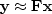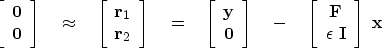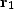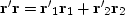Next: ITERATIVE METHODS Up: Time domain versus frequency Previous: Unknown filter

### Unknown input: deconvolution with a known filter

For the unknown input problem we put the known filter ft in a matrix of downshifted columns.Our statement of wishes is now to find xt so that.We can expect to have trouble finding unknown filter inputs xt when we are dealing with certain kinds of filters, such as bandpass filters. If the output is zero in a frequency band, we will never be able to find the input in that band and will need to prevent xt from diverging there. We do this by the statement that we wish,whereis a parameter that is small and whose exact size will be chosen by experimentation. Putting both wishes into a single, partitioned matrix equation gives(28)
To minimize the residualsand,we can minimize the scalar.This is(29)
We have already solved this minimization in chapterin the frequency domain (beginning from equation ()).

Formally the solution is found just as with equation (26), but this solution looks unappealing in practice because there are so many unknowns and because the problem can be solved much more quickly in the Fourier domain. To motivate ourselves to solve this problem in the time domain, we need either to find an approximate solution method that is much faster, or to discover that constraints or time-variable weighting functions are required in some applications.

## EXERCISES:

1. Try other lags in (19) such as (0,1,0)' and (0,0,1)'. Which works best? Why?

1Next: ITERATIVE METHODS Up: Time domain versus frequency Previous: Unknown filter
Stanford Exploration Project
10/21/1998